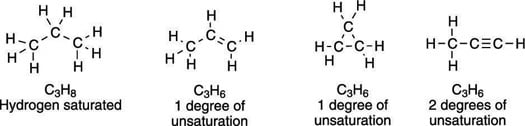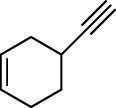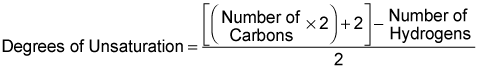##### Organic Chemistry I For Dummies, 2nd EditionKnowing the number of degrees of unsaturation in a molecule is useful because this number is related to how many multiple bonds or rings are present in an unknown compound. (This morsel of information becomes very useful when you want to determine the structure of an unknown compound.)

## One double bond, one degree of unsaturation

The degrees of unsaturation in a molecule are additive — a molecule with one double bond has one degree of unsaturation, a molecule with two double bonds has two degrees of unsaturation, and so forth.

Just as the formation of a double bond causes two hydrogens to be lost, the formation of a ring also results in the loss of two hydrogens, so every ring in the molecule also adds one degree of unsaturation.

For every triple bond, two degrees of unsaturation are added to a molecule, because a molecule must lose four hydrogens to make a triple bond. Some examples of three-carbon molecules with different numbers of degrees of unsaturation are shown here.The degrees of unsaturation for three-carbon molecules
To determine the number of degrees of unsaturation for any arbitrary structure, you sum all the individual elements of unsaturation in the molecule. The next figure shows a molecule that consists of one ring, one double bond, and one triple bond. This molecule, therefore, has four degrees of unsaturation because the double bond and the ring each add one degree of unsaturation, and the triple bond adds two degrees, for a total of four.A molecule with four degrees of unsaturation

## The goal: Using a molecular formula

More important than determining the number of degrees of unsaturation from a molecular structure is being able to determine the number of degrees of unsaturation from a molecular formula. The number of degrees of unsaturation can be determined from the molecular formula using the following equation.With this equation, the number of degrees of unsaturation can be determined for any hydrocarbon whose molecular formula is known. (For compounds whose structure and formula are not known, chemists use an instrumental technique called mass spectrometry to determine the molecular formula of the compound.)

But what about molecules that contain atoms other than hydrogen and carbon? In such cases, you need to convert these multi-atom molecular formulas into equivalent formulas that contain just carbon and hydrogen so they can be plugged into the preceding equation. To do so, you use the following conversion factors:

• Halogens (F, Cl, Br, I): Add one hydrogen to the molecular formula for each halogen present.

• Nitrogen: Subtract one hydrogen for each nitrogen present.

• Oxygen or sulfur: Ignore.

For example, to determine the number of degrees of unsaturation in the formula C8H6F3NO2, you first make the proper substitutions for all atoms that are not hydrogen and carbon. Fluorine is a halogen, so you add three hydrogen atoms to the molecular formula (one for each F). The molecule contains one nitrogen, so you subtract one hydrogen from the molecular formula. The two oxygens in the molecule you ignore. This gives a reduced equation of C8H6+3–1 = C8H8. In other words, both the formula C8H6F3NO2 and the formula C8H8 have identical numbers of degrees of unsaturation. Plugging this reduced formula into the preceding equation gives five degrees of unsaturation for the molecular formula C8H6F3NO2.